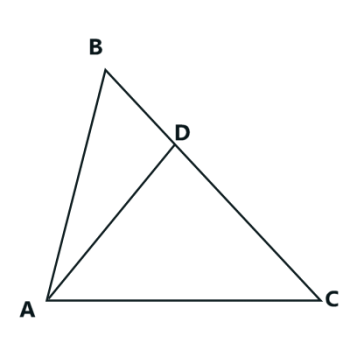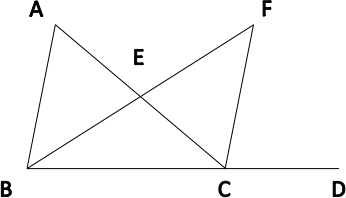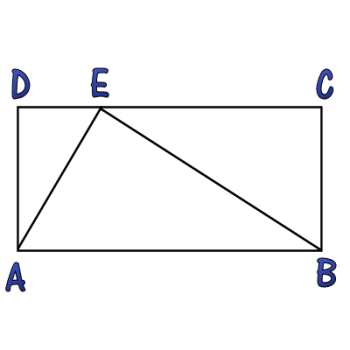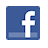Games
Problems
Go Pro!

# Three Triangles

Pro Problems > Math > Geometry > Triangles

## Three Triangles

The angles in a triangle have measures x, x + 10, and y.

The angles in a second triangle have measures x + y, 40, and 50.

The angles in a third triangle have measures x – y, 40, and z.

What is the value of z?

Presentation mode
Problem by Mr. Twitchell

## Solution

In order to make it feasible for teachers to use these problems in their classwork, no solutions are publicly visible, so students cannot simply look up the answers. If you would like to view the solutions to these problems, you must have a Virtual Classroom subscription.Assign this problem

## Similar Problems

### Split Triangle

Segment AD is drawn in triangle ABC, with D on side BC. Given the following information, determine the measure of angle ADB.measure of angle BAD = x
measure of angle CAD = 2x
measure of angle ADC = 2y
measure of angle ABD = y
measure of angle ACD = x + y

### Triangle in a Square

A triangle is placed on the interior of a square so that two of the triangle's vertices lie on two adjacent vertices of the square, and the third vertex lies on the opposite side,
1
3
of the way between the two endpoints.

If the perimeter of the triangle (in linear units) is equal to the area of the triangle (in square units), what is the length of the side of the square?

### Angle Measure

Line segment AB is intersected by segment DC, with D on AB, between A and B.

In triangle ADC, m∠ADC = 30º + x and  m∠DAC = 50º

In triangle ABC, m∠ACB = 115º + x

In triangle CDB, m∠CDB = 140º + x

Find m∠CBD.

### Angle Bisector

In the diagram shown,m ∠DCF = 80
m ∠ACF = 30
m ∠FBD = 50.

If segment BF bisects  ∠ABD, find m ∠BAC.

### Angles in a Triangle

In a triangle, the measure of the largest angle is 12 less than the sum of the measures of the other two angles. The largest angle is also 52 more than the twice the middle angle decreased by three times the smallest angle. What is the largest angle measure?

### Triangle and RectangleIn the diagram displayed, the triangle splits the rectangle into three similar triangles. Find the value of AD2 divided by the product of DE and EC.

### Two Triangles with Angle Measures

In a triangle, the angles measures are: 3x + 44, x, and 2x - 20. In a second triangle, two of the angle measures are x + 20 and 2x - 5. What is the measure of the third angle?

The angles in a triangle have measures x2 - 5, 2x + 18, and x + 37. What is the measure of the largest angle in the triangle?

### Triangle Variables

An isosceles triangle is a triangle in which two of the angles have the same measure. In an isosceles triangle, the angle measures are 30 + x, 62 - x, and 10 + 2x + y. What are the possible numeric values for the measures of the angles?

### Triangle in a Square

Triangle ABC is inside square ABDE, and C lies along side DE. If the area of the triangle is 24 square units, what is the length of AB?

Three Two-Digit Angle Measures, Arithmetic Angles, Angle Riddle, Forty-five and Thirty# Featured Resources on This Site

Create Christmas ornaments and more
Print fun mazes in many different shapes

# Blogs on This SiteReviews and book lists - books we love!The site administrator fields questions from visitors.Like us on Facebook to get updates about new resources